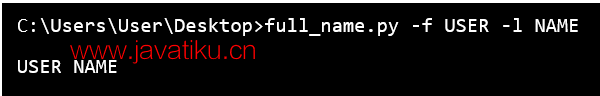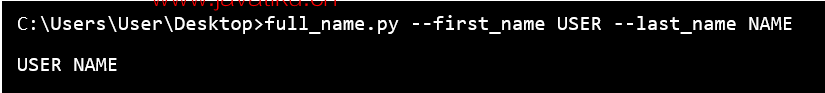# Tkinter 教程-Python中的Getopt模块Getopt模块是用于处理基于UNIX getopt()函数规范的命令行选项的分析器。它主要用于分析类似于sys.argv的参数序列。我们还可以将这个模块理解为帮助脚本分析sys.argv中的命令行参数的工具。该模块的工作方式类似于C编程语言中的getopt()函数，用于分析命令行参数。

## Python Getopt函数

``getopt.getopt ( args , options , [ long_options ] )  ``

getopt.getopt()模块接受以下参数。

args: args是要传递的参数列表。

options: options是要识别的选项字母的字符串。需要参数的选项应该带有冒号(:)。

long_options: 这是包含长选项名称的字符串列表。需要参数的选项应该带有等号(=)。

``````-   - a
-   - bvalue
-   - b value
-   -- noargument
-   -- withargument=value
-   -- withargument value  ``````

## Getopt()函数的参数

getopt()模块函数有三个参数：

• getopt()模块的第一个参数是要分析的参数的分类。通常来自sys.argv[1:]（程序名称在sys.argv中被忽略）的参数序列。
• 第二个参数是用于单字符选项的选项定义的字符串。如果任何选项需要参数，它的字母后面带有冒号[:]。
• getopt()模块函数的第三个参数是长格式选项的名称序列。长格式选项的名称可以由多个字符组成，例如：-- noargument或-- withargument。在参数序列中选项的名称不能包括" -- "前缀。如果任何长格式选项需要参数，其名称应带有等号(=)。

## 短形式选项：

``````import getopt     #importing the getopt module

print getopt.getopt ( [ ' -a ' , ' -bval ' , ' -c ' , ' val ' ] , ' ab:c: ' )
\$ python getopt_short.py

( [ ( ' -a ' , ' ' ) , ( ' -b ' , ' val ' ) , ( ' -c ' , ' val ' ) ] , [ ] ) ``````

## Getopt()中的长格式选项

``````import getopt                               # importing getopt () module
print getopt.getopt ( [ ' -noarguement ' , ' -witharguement ' , ' value ' , ' -witharguement2 = another ' ] , '  ' , [ ' noarguement ' , ' witharguement = ' , ' witharguement2 = ' ] )
\$ python getopt_long.py
( [ ( ' -noarguement ' , ' ' ) , ( '?witharguement ' , ' value ' ) , ( ' -witharguement2 ' , ' another ' ) ] , [ ] )  ``````

### 示例1：

``````import sys
# importing getopt module
import getopt

def full_name ( ):
first_name = None
last_name = None

argv = sys.argv [ 1: ]

try:
opts , args = getopt.getopt ( argv, " f:l: " )

except:
print ( " Error " )

for opt , arg in opts:
if opt in [ ' -f ' ]:
first_name = arg
elif opt in [ ' -l ' ] :
last_name = arg

print ( first_name + " " + last_name )

full_name ( )   ``````### 示例2：

``````import sys
import getopt                                           # import getopt module

def full_name ( ):
first_name = None
last_name = None

argv = sys.argv [ 1: ]

try:
opts , args = getopt.getopt ( argv , " f:l: " ,  [ " first_name = " , " last_name = " ] )

except:
print ( " Error " )

for opt , arg in opts:
if opt in [ ' -f ' , ' --first_name ' ] :
first_name = arg
elif opt in [ ' -l ' , ' -- last_name ' ] :
last_name = arg

print ( first_name + " " + last_name )

full_name ( )   ``````